# AP Physics 1 Question 369: Answer and Explanation

### Test Information

Question: 369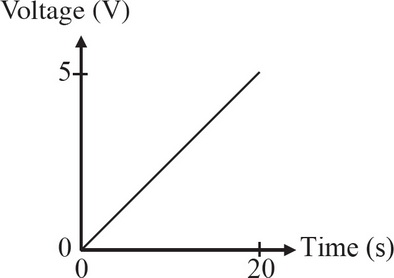7. A circuit consists of a 500 Ω resistor connected to a variable voltage source. The voltage is increased linearly from 0 V to 5 V over a period of 20 s, as shown in the graph above. Which of the following graphs corresponds to the power dissipated by the resistor as a function of time?

• A.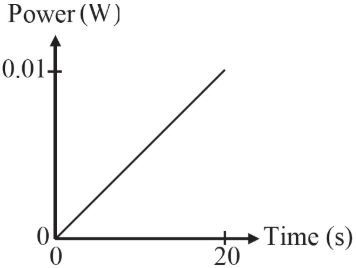• B.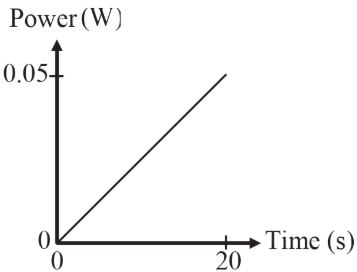• C.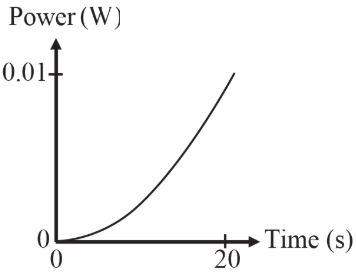• D.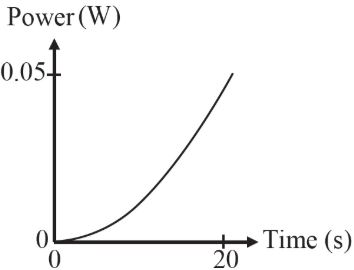Explanation:

D

At 20 s, the voltage across the resistor is 5 V, so the current is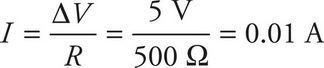The power at this time is given by

P = IΔV = (0.01 A)(5 V) = 0.05 W

The shape of the graph can be obtained by combining these two equations: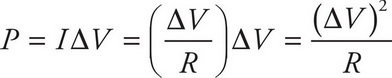which shows that the power dissipated by the resistor will increase quadratically with the voltage.